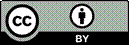Article citation information:

Gherman, L., Rotaru, C., Pearsica, M. Electromagnetic launch system based on a Halbach array configuration. Scientific Journal of Silesian University of Technology. Series Transport. 2018, 101, 59-66. ISSN: 0209-3324. DOI: https://doi.org/10.20858/sjsutst.2018.101.6.

Laurian GHERMAN, Constantin ROTARU, Marian PEARSICA

ELECTROMAGNETIC LAUNCH SYSTEM BASED ON A HALBACH ARRAY CONFIGURATION

Summary. The paper analyses a new configuration of an electromagnetic launch system (EMLS) able to accelerate a 1-kg mass projectile at a muzzle velocity of 3,000 m/s with an acceleration length of 10 m. The analyses consist of a mathematical calculation of the value of acceleration force necessary to achieve the project objectives. Based on these results a new configuration of an EMLS is presented. The projectile is accelerated by the Lorentz force where the magnetic field is created by permanent magnets made of neodymium iron boron (NdFeB). The permanent magnets are arranged in a cylindrical Halbach array with a uniform field inside. The copper wire coils have a special design, which produce a large acceleration Lorentz force with a current under 1,000 A. A short comparative0 analysis shows the advantages of this new solution compared with well-known accelerators such as railguns and coilguns. In the next part of the paper, we present the results of a simulation of this configuration with an interactive software package based on the finite element method used to analyse and solve three-dimensional electromagnetic field problems and simulate the movement of the projectile. These results confirm the advantages of this new configuration and open the way to test the system under laboratory conditions. At the end of the paper, conclusions are presented. This new EMLS, based on Halbach array configuration, can be used as a catapult for UAVs, airplanes or missiles, as a mass driver for small satellites or as a gun, which is a better solution than railguns or coilguns.

Keywords: Lorentz force; Halbach array; electromagnetic catapult; finite element method

1. INTRODUCTION

In this paper we present the theoretical results obtained during a project aimed to explore new ways to accelerate a mass using electromagnetic energy. The objective of the project is to accelerate a projectile with a mass of 1 kg from 0 to 3,000 m/s using 10 m of acceleration length. According to this objective, the kinetic energy of the projectile is:(1)

If we assume the initial speed to be 0 and the acceleration force acting on projectile to be constant, then the value of the force is: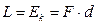(2)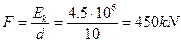(3)

According to our project objectives, the accelerating force acting on the projectile is a Lorentz force. The equation of the Lorentz force, based on current intensities, which are sometimes presented as a Laplace force, IS as follows: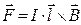(4)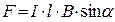(5)

where α is the angle between vectorsand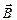.

We assume the magnetic field to be perpendicular to the current caring wire (α=900).

We analysed the existing railgun and coilgun electromagnetic systems and observed a few similarities. Both use the Lorentz force to accelerate the projectile, but the most important observation is that the moving part is the current caring wire and the magnetic field is created by the stator.

This configuration is, according to the books, located where the magnetic field is static and the wire is moving. In our project, we explore a new way where the wire is the stator, and the projectile creates the magnetic field. The magnetic field is created by permanent magnets made of neodymium iron boron (NdFeB) N52 with residual flux density Br=1.45-1.48 T. For our system, we choose a ring with a Halbach array arrangement of eight permanent magnets in order to augment the magnetic field inside the ring. For the best results, we choose arrangements of permanent magnets to create a uniform magnetic field inside the ring.

We expect non-uniformities of magnetic fields created by end effects, while only taking them into consideration during simulations. For simplicity in terms of the mathematical calculation of the Lorentz force created, we assume the magnetic field to be uniform and only inside the ring.

In Figure 1, we present the simulation of the arrangement of permanent magnets and directions of magnetization in order to obtain the uniform magnetic field inside the ring. The Maxwell interactive software package, which uses the finite element method (FEM), was used to analyse and solve three-dimensional electromagnetic field problems, as well as simulate the magnetic field created by the circular Halbach array. The residual flux density used for calculation purposes is Br=1.45 T.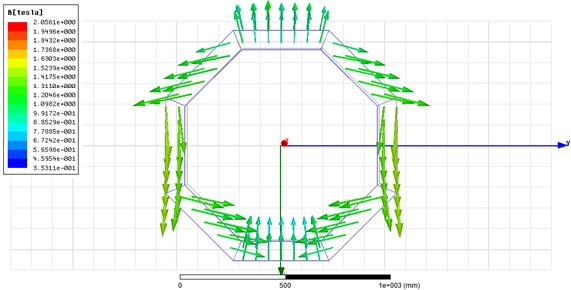Fig. 1. Halbach array configuration ring

In Figure 2, we present the simulation result of a Halbach array ring made from permanent NdFeB N52 magnets. According to theory, the magnetic field lines inside the ring are linear, starting from the bottom part of the ring to the upper part of the ring.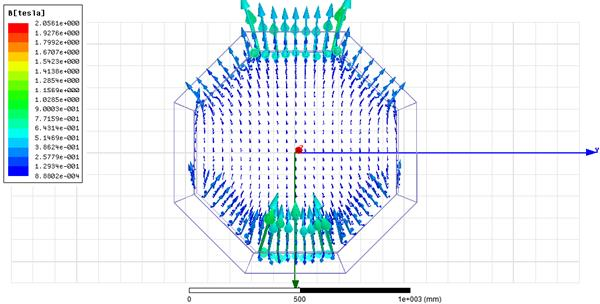Fig. 2. Magnetic field lines inside the Halbach array ring

As we expected, the magnetic field density is stronger when in close range of the permanent magnets.

As the current carrying wire must be placed in an area with a strong magnetic field, we should calculate the dimensions of this area. Using the simulation software, we obtained the following results.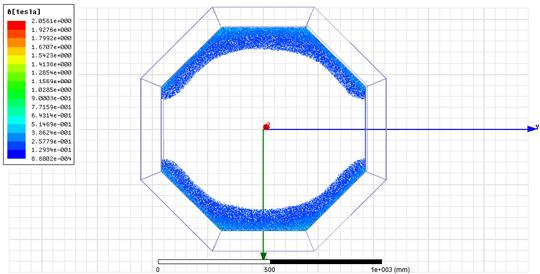Fig. 3. The area inside the ring with the strongest magnetic fields

According to the simulation results displayed in Figure 3, the best area to place the current carrying wire is inside the area shown with blue colour. After that boundary, the density of the magnetic field drops below 0.1 T a value with no significant impact for our project.

We used simulation software to calculate the dimensions of the Halbach array ring in order to obtain an area big enough to accommodate many wires. The dimensions are as follows: inner diameter=1,000 mm, outer diameter=1,200 mm and height of the permanent magnets=120 mm. At these dimensions, we obtained a rectangle cross-sectional area for wires with the following dimensions: height=120 mm and length=50 mm.

In order to design the stator coil, we should make some calculus. To keep our calculus simple, let us assume that the magnetic field density has the same value, i.e., 1.45 T, for every wire inside the magnetic field lines. We will obtain more precise results from simulation. From the Lorentz force equation, we have:(6)

If we consider the length of a wire as 1 m, then I=3.1∙106 A. This value is close to the value of the current used in the railgun system. For our project, we are looking for a design that uses a current with a low value. According to Equation 6, we can lower the value of current intensity I by increasing the value of wire length l. As the dimensions of the Halbach array ring cannot be increased, we choose to design a special wire coil with N turns. The number N depends on the size of the wire. We sought a copper wire able to sustain a fusing current calculated per Onderdonk for 32 ms up to 1,000 A.  From the table with American wire gauges, we find AWG 19 with a diameter of 0.912 mm. At this dimension of wire, we can accommodate N=4,600 turns in the rectangle cross-sectional area obtained via simulation.

According to our calculus with assumptions, the value of the necessary intensity of the current is I=673.91 A. Theoretically, it is possible, considering the made assumptions, to achieve the project objective using a direct current with a value of intensity less than 1,000 A.

The next step is to design a special form of winding because we cannot use an ordinary coil. The direction of current in the lower part of the ring must be the same as in the upper part of the ring. In an ordinary coil, the current is in opposite direction.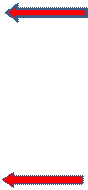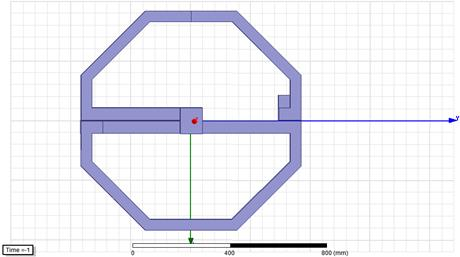Fig. 4. The winding with the direction of current

For our project, the winding has the shape of the number 8 in order to obtain the same direction of current on both sides of winding. We use the centre part of the Halbach array ring to connect both sides of winding because, in that region, the density of the magnetic field is at lowest level.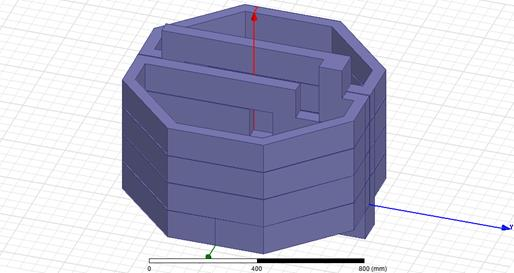Fig. 5. The four stages of winding

For our project, we choose only four stages of winding to simulate the acceleration length. The dimension of this acceleration length is 0.480 m. According to the presented assumptions, we need a speed of 657 m/s after 0.480 m to obtain a muzzle velocity of 3,000 m/s after 10 m of acceleration.

To summarize, theoretically, we need a current with intensity of I=673.91 A to obtain an acceleration force of F=450 kN if we use the presented design. The next chapter of our paper presents the results of simulations of this design without assumptions used for theoretical calculation purposes.

2. SIMULATIONS

First, we built the simulation model with the same dimensions as we used to calculate the theoretical values of current intensity I, acceleration force F and muzzle velocity v.

As the simulation software calculates the Lorentz force without assumptions, we expect a slightly different value for the intensity of current. The value of force must to be the same as when we calculate the same muzzle velocity. The simulation model is displayed in Figure 6.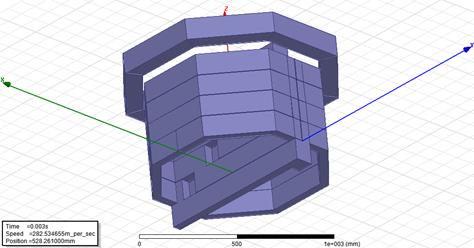Fig. 6. The simulation model

The moving part is the Halbach array ring, which is created inside the magnetic field. Inside the ring is the winding, which is the acceleration part of the system. The mass of the ring is set at 1 kg. Figure 6 presents the position of the ring after acceleration.

We simulate the movement of the Halbach array ring for different values of intensity of the current through wires and choose to present the values obtained for I=500 A. Figure 7 presents the variation of ring speed and the ring position in time. The system accelerates the ring up to a maximum speed of 703.23 m/s.

The muzzle velocity of the ring is 669.85 m/s, when the bottom parts of the ring pass the limit of the acceleration length. This value is close to the calculated value of 657 m/s. The acceleration time is 1.44 ms. In Figure 7, the time variation of ring speed is presented with a dotted line, while the position of the ring in time is presented with a dashed line.

Figure 8 displays the time variation of the Lorentz force acting on the ring with a dotted line, while the position of the ring in time is with a dashed line. The force achieves a maximum value of 911.37 kN when the ring is in the last stage of accelerated winding.Fig. 7. The muzzle velocity

After that position, the value of force decreases because the magnetic field of the Halbach array ring does not intersect with the winding; and, when the bottom part of the ring departs from the acceleration length, the non-uniformities of the magnetic field created by the end effects create the deceleration force. This force has a small influence on velocities because the ring spends only 0.07 ms in this region.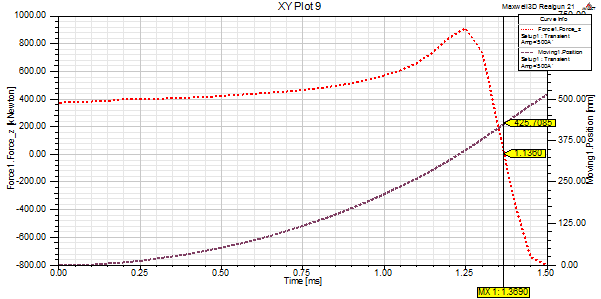Fig. 8. The Lorentz force variation in time

3. CONCLUSION

In this paper, we have theoretically proven, through simulation, the possibility to obtain high muzzle velocities of a projectile using a current with a low intensity of 500 A. As the ring is outside the winding, it is possible to accelerate an object mechanically connected with the ring.

If we want to decrease the acceleration length, we can use two or more rings with two or more acceleration windings. The object can be attached to these rings in order for them to be accelerated. If the acceleration mass is increased, the intensity of the current should be increased in order to obtain the desired muzzle velocity.

### Acknowledgements

The paper was prepared under the project “Microlauncher Based on a Detonation Engine – MILADEE”, Contract 174/2017, part of the Romanian Space Agency’s STAR programme.

References

1.             Fair H.D. 2007. “Progress in Electromagnetic Launch Science and Technology”. IEEE Trans. on Magnetics 43(1): 93-98.

2.             Balikci A., Z. Zabar, L. Birenbaum, D. Czarkowski. 2007. “On the Design of Coilguns for Super-velocity Launchers”. IEEE Trans. on Magnetics 43(1): 107-110.

3.             Polzin K.A., J.E. Adwar, A.K. Hallock. 2013. “Optimization of Electrodynamic Energy Transfer in Coilguns with Multiple, Uncoupled Stages”. IEEE Trans. on Magnetics 49(4): 1453-1460.

4.             Wenbo L., W. Yu, G. Zhixing, Y. Zhongming, C. Weirong. 2013. “Connection Pattern Research and Experimental Realization of Single Stage Multipole Field Electromagnetic Launcher”. IEEE Trans. on Plasma Science 41(11): 3173-3179.

5.             Su Z. et al. 2015. “Investigation of Armature Capture Effect on Synchronous Induction Coilgun”. IEEE Transactions on Plasma Science 43(5): 1215-1219. DOI: 10.1109/TPS.2015.2410302.

6.             Abdalla M.A., H.M. Mohamed. 2016. “Asymmetric Multistage Synchronous Inductive Coilgun for Length Reduction, Higher Muzzle Velocity, and Launching Time Reduction”. IEEE Transactions on Plasma Science 44(5): 785-789. DOI: 10.1109/TPS.2016.2543500.

7.             Perotoni M.B., M. Mergl, V.A. Bernardes. 2017. “Coilgun Velocity Optimization with Current Switch Circuit”. IEEE Transactions on Plasma Science 45(6): 1015-1019. DOI: 10.1109/TPS.2017.2700789.

Received 23.07.2018; accepted in revised form 02.11.2018